# Sampling Methods

Posted by Gwan Siu on March 29, 2018

## 1.Introduction

In this article, I will discuss some sampling methods: Inverse Samling, Reject-Accept Sampling, Important Sampling, and its application partical filter.

Why do we need sampling? In many inference tasks (such as computing marginal probability $P(x)$, computing the partition function $A(\theta)$, or find the expectation of arbitrary function), we are interested in quatities that in a sense sum over the configuration of a true distribution

In such problems, true distribution in closed-form is hard to be obtained and this integral operator in high-dimensional space is intractable. However, if we can sample from this distribution, approximate inference is possible by using a sample-based method of $p(x)$ because large number theory. Here, we have $N$ sample from the true distribution and the expectation of this true distribution can be approximated by

this is essentail idea behind of the Monte Carol, which gives us a stochastic representation of a potentially complex function. This representation can then be used to compute the marginals and expectations in which we are interested. Actually, the approximate are asymptotically exact (they get close to the true $E_{p}[f(x)]$ as $N\rightarrow \infty$), and flexible for any distribution. However, there are key non-trival challenges that must be overcome:

1. How exactly do we draw from them from complex distributions?
2. Not all samples are equally useful.
3. How do we knwo we’ve draw enough samples.

## 2. Inverse sampling (naive)

The idea behind inverse sampling is very ituitive: to transform uniform samples into samples from a different distribution. That is, by somehow drawing from an uniform distribution(because CDF is range from 0 to 1), we make it possible to draw from the other distribution. The procedure of inverse sampling is illustrated as:The assumption of inverse sampling is that CDF must be invertiable!

The algorithm of inverse sampling is:

1. get a uniform sample $\mu$ from U (0,1)
2. obtain the sample $x$ through $x=F^{-1}(\mu)$ where F is the CDF distribution we desire.
3. repeat.

Why does inverse sampling work?

To be note that:

What’s the ditribution does the random variable $y=F^{-1}(\mu)$ follow?

The CDF of y is $p(y\leq x)$. Since the CDF is monotonic, we can obtain without loss of generity:

Thus we get the CDF and hence the pdf from which we want to sample.

Limitation of Inverse Sampling

Not all the pdf has analytical CDF, for example, gaussian ditribution. In addition, for some complex distributions, the inverse CDF may be complicated and it is hard to do inverse sampling.

## 2. Rejection Sampling

### 2.1 Basic Rejection Sampling

The basic idea is come up with von Neumann. If you have a complex function $p(x)$ you are trying to sample from, whose maximum value and minimum value are knowbm, basically accept the sample by generating a uniform random number at any $x$ and the range of $p(x)$, and accepting it if the value is below the value of the function at that $x$. The procedure of basic sampling is illustrated as: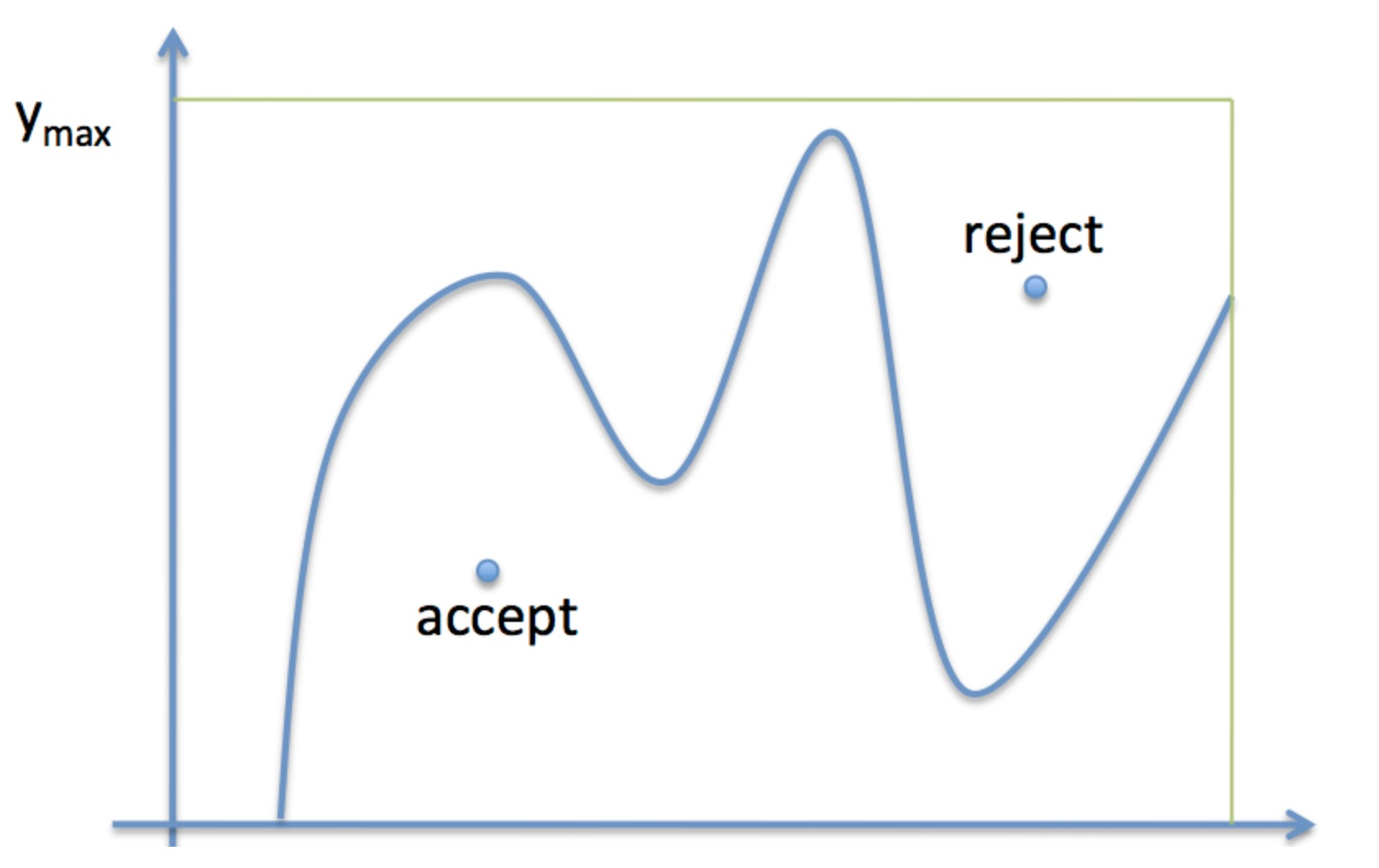The procedure of basic rejection sampling:

1. Draw $x$ uniformly from $[x_{min}, x_{max}]$.
2. Draw $y$ uniformly from $[0, y_{max}]$.
3. If $y\leq f(x)$, accept. Else, reject.
4. Repeat.

The intuitive explaination: This works as more samples will be accepted in the regions of $x$-space where the function $f$ is higher: indeed they will be accepted in the ratio of the height of the function at any given $x$ to $y_{max}$. From the perspective of probability interpretation, the accept-to-total ratio reflects the probability mass in each x silver.

Code of Basic Rejection Sampling

## target function

f = lambda x: np.exp(-x)
#f = lambda x x**2

## domain limits
xmin = 0 # lower bound of feasiable domain
xmax = 10 #upper bound of feasiable domain

## range limit for y
ymax=1
#ymax = 100

N = 10000 #the total of samples
accept =0 #count the total number of acceptance
samples = np.zeros(N)
count = 0

while (accept <N):

x = np.random.uniform(xmin,xmax)
y = np.random.uniform(0, ymax)

if y<f(x):
samples[accept]=x
accept += 1
count +=1

print('Count: ', count, ', Accepted: ', accept)

hinfo = np.histogram(samples, 30)
plt.hist(samples, bins=30, label='Smaples')
print(hinfo)
xvals = np.linspace(xmin, xmax, 10000)
plt.plot(xvals, hinfo*f(xvals), 'r', label='f(x)')
plt.grid('on')
plt.legend()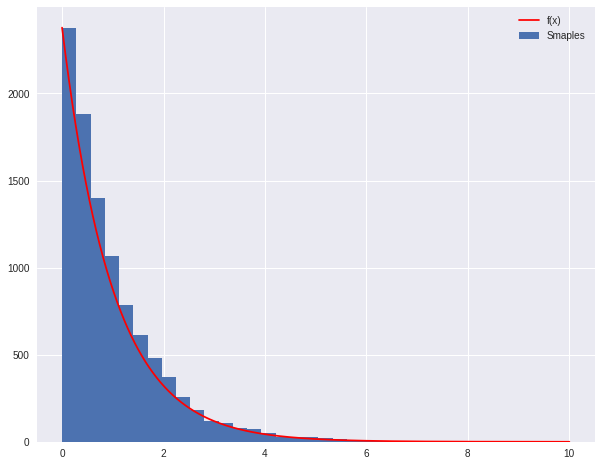### 2.2 Modified Reject Sampling

Low accetance rate is one deterministic limitation of basic rejectioin sampling. Now let’s consider a case where

• The target distribution $p(x)$ is difficult to sample from.
• The unnormalized distribution $\hat{p}(x)=\frac{1}{Z}p(x)$ is easy to evaluate. Note that this alone does not make $p(x)$ amensable to sampling.
• The proposal distribution $q(x)$ is a distribution that we can easily sample from (e.g. uniform or normal)
• $k$ is chosen constant such that $kq(x)\geq \hat{p}(x)$ for all $x$. This is called the comparison function.

Procedure

1. Sample $x_{0}$ from $q(x)$.
2. Sample a number $\mu_{0}$ from the uniform distribution over $[0, kq(x_{0})]$
3. Reject the sample if $\mu_{0}>\hat{p}(x_{0})$ and retain the sample otherwise.

Note that the probability of accepting sample $x_{0}$ is $\frac{\hat{p}(x_{0})}{kq(x_{0})}$. Pictorially for a univariate case, this process is akin to sampling uniformly any point in the area under the $kq(x)$ curve and accepting only if it does not land in the gray region.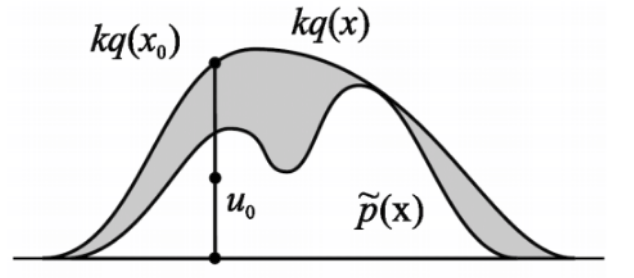Correctness

Formally show that this procedure samples correctly from $p(x)$. Firstm we observe that the procedure selects a particular $x$ with density proportional to $q(x)\cdot \frac{\hat{p}(x)}{kq(x)}$. Then, the sampling mechanism generates samples according to a distribution $p_{s}(x)$ which is equal to

Limitation

If the proposal distribution $q(x)$ is not chosen well (i.e. differs greatly from $p(x)$), then even an optimally chosen $k$ can result tin a huge rejection region. This implies a large waste of samples that will be rejected. Even if distributions seem similar, in high dimensions this rejection volumn can be very large. For example, $d-$dimensional gaussians

for $d=1000$ and $\sigma_{q}$ only 1 percent bigger than $\sigma_{p}$ result in an acceptance rate of only $\approx \frac{1}{2000}$. One potential way to fix this is to use adaptive rejection sampling, which covers $\tilde{p}$ with an envelope of piecewise functions instead of one proposal distribution $q$ but this gets rather complicated.

The code of modified rejection sampling


p = lambda x: np.exp(-x)  # our distribution
g = lambda x: 1/(x+1)  # our proposal pdf (we're thus choosing M to be 1)
invCDFg = lambda x: np.log(x +1) # generates our proposal using inverse sampling

# domain limits
xmin = 0 # the lower limit of our domain
xmax = 10 # the upper limit of our domain

# range limits for inverse sampling
umin = invCDFg(xmin)
umax = invCDFg(xmax)

N = 10000 # the total of samples we wish to generate
accepted = 0 # the number of accepted samples
samples = np.zeros(N)
count = 0 # the total count of proposals

# generation loop
while (accepted < N):

# Sample from g using inverse sampling
u = np.random.uniform(umin, umax)
xproposal = np.exp(u) - 1

# pick a uniform number on [0, 1)
y = np.random.uniform(0,1)

# Do the accept/reject comparison
if y < p(xproposal)/g(xproposal):
samples[accepted] = xproposal
accepted += 1

count +=1

print("Count", count, "Accepted", accepted)

# get the histogram info
hinfo = np.histogram(samples,50)

# plot the histogram
plt.hist(samples,bins=50, label=u'Samples');

# plot our (normalized) function
xvals=np.linspace(xmin, xmax, 1000)
plt.plot(xvals, hinfo*p(xvals), 'r', label=u'p(x)')
plt.plot(xvals, hinfo*g(xvals), 'k', label=u'g(x)')

# turn on the legend
plt.legend()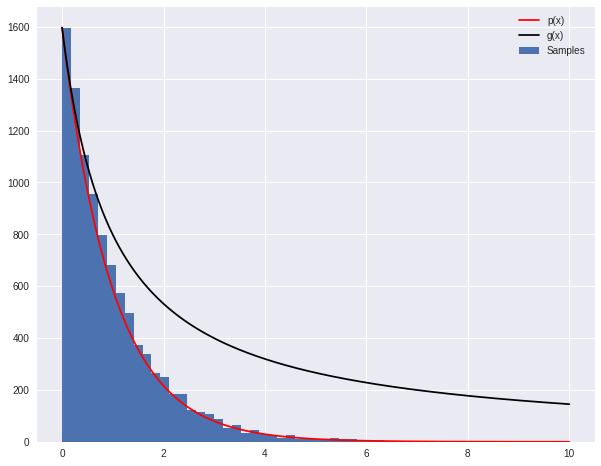## 3. Important Sampling

Different from reject sampling, import sampling does not has any rejection action. To replace with rejection action, important sampling adopt the approach of weighted sample. Specifically, suppose we want to evaluate expectations using samplings from a complicated probability distribution. We assume that

• $p(x)$ is hard to sample from but easy to evaluate
• $q(x)$ is easy to sample from
• $f(x)$ is a function we want to evaluate in expectation: $E_{p}[f(x)]$
• $q(x)>0$ whenever $p(x)>0$ or $q$ dominates $p$.

Procedure (unnormalized important sampling)

1. Draw $M$ samples $x_{m}$ from $q(x)$.
2. Determine weights $w_{m}$ for samples equal to the likelihood ratio $w_{m}=\frac{p(x_{m})}{q(x_{m})}$
3. Compute expectation as:

We call it unnormalized because these weights are likelihood are likelihood ratios, so there is no reason that they need to sum to 1. However, it gives us a first approximation to the true distribution.

Note that this does not give us sample from the target distribution but we can prove corretness for the expected value estimate

The key step is that third equality where we can approximate the integral assuming $x_{m}$ are drawn from $q(x)$ which they acyually are in the procedure.

Normalized important sampling

Here we no longer assume that we know $p(x)$ and instead only know it up to a constant factor $\hat{p}(x)=\alpha p(x)$. This is a common situation, such as when we want a conditional probability when we know the joint $p(x, e)$ but not the marginal $p(e)$. In this case, the samplilng procedure is

Procedure

1. Draw $M$ samples $x_{m}$ from $q(x)$.
2. Calculate ratios $r_{m}$ for samples equal to $r_{m}=\frac{\hat{p}(x_{m})}{q(x_{m})}$.
3. Compute expectation as

We observe first that

Again the key step is the fourth equality were we approximate both numerator and denominator using samples drawn form $q(x)$. Here we observe that $\sum_{m}w_{m}=1$, hence why we call it the normalized version. The key takeaway is that we don’t need to know the normalization constant for the target distribution.

Comparison between normalized and unnormalized

On finte samples. The unnormalized version gives an unbiased estimator of the true expectation while the normalized version gives a biased estimator of the true expectation. However, the variance of the normalized version is generally lower in practice.

Limitation

These importance sampling approaches are based on likelihood weighting, which is simple to operate but still might be inaccurate in some peculiar scenarios. Again the core issue is when our proposal and target distributions are not similar enough. Consider the following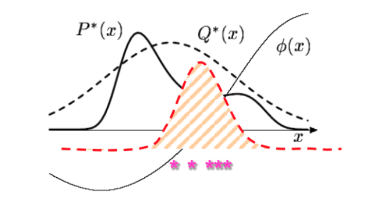Essentially, what importance sampling is trying to do is to weight the samples such that they reflect relative importance to each other. The hope is that even if regions where $P$ has high density are low probability regions in $Q$ , the weighting on these samples will be high enough to offset the fact we won’t see many samples in this region. Similar to the arguments against direct sampling, if $Q$ has really thin tails where $P$ has high probability (and where the means are going to actually be located around), we may simply never see enough samples from this region. We might need an extraordinary number of samples to offset this, meaning most of our samples are wasteful as they are very low importance. In terms of a sampling algorithm, this is compounded by the fact that usually the stopping condition is when the estimate for the mean of $f(x)$ starts to converge. However, in the scenario we described, it’s possible to have a stable estimate even if all the samples are coming from low probability regions of $P$. Thus, the algorithm will stop even though the mean estimate is very inaccurate. There are a couple of potential solutions to this problem.

1. We could use heavy-tailed proposal distributions. However, this has the disadvantage of inefficiently drawing a lot of wasted samples with low importance.

2. We can use weighted re-sampling.

Weighted Re-sampling

1. Draw $N$ samples from $q:X_{1},…,X_{N}$
2. Construct wegihts $w_{1},…,w_{N}$ equal to $w_{m}=\frac{P(x_{m})Q(x_{m})}{\sum_{m}P(x_{m})Q(x_{m})}=\frac{r_{m}}{\sum_{m}r_{m}}$.
3. Sub-sample examples $x$ from $X_{1},…,X_{N}$ with probability $w_{1},…,w_{N}$ with usually $N^{\prime}\geq\geq N$.

This is a sense amplifies the high importance samples while diminishes the low importance samples.

(Example: Expectation)

Choose a distribution $g(x)$, which is close to the function $f(x)$, but which is simple enough so that it is possible to generate random $x$-values from this distribution. The integral can now be re-written as:

Therefore if we choose random number $x_{i}$ from distribution $g(x)$, we obtain:

Let $w(x_{i})=\frac{f(x_{i})}{g(x_{i})}$, the formulation can be rewritten:

Now the variance(error) of monte carol is that:

where $N$ is the sample size.

With the important sampling this formula has now changed to

Our goal is to minimize the $V_{g}[\omega(x)h(x)]$.

As a somewhat absurd notion, this variance should be set to zero, if

which leads to (since $g(x)$ is density thus we need normalization):

Actually, the expection is what we expect to estimate. Let’s ignore the denominator, this formula tell us that to achieve low variance, we must have $g(x)$ large where the product $f(x)h(x)$ is large. Didirectly, maximizing the latter in some fashion was our original intuition.

Or from another perspective, $\frac{f(x)}{g(x)}$ ought to be large where $h(x)$ is large. This means that, as we say earlier, choose more samples near the peak.

In detail, We have a $f$ that we might or might not know. We have a pdf $g$ which we choose to be higher than $f$ at the points where hh has peaks. Now what we are left to do is to sample from $g$, and this will give us an oversampling at the place hh has peaks, and thus we must correct this there by multiplying by weights $w=\frac{f}{g}<1$ in thse places.

Be careful to choose $g(x)$ appropriately, it should have thicker tails than $f$, or the ratio $\frac{f}{g}$ will be too big and count contribute too much in the tails. All of these considerations may be seen in the diagram below: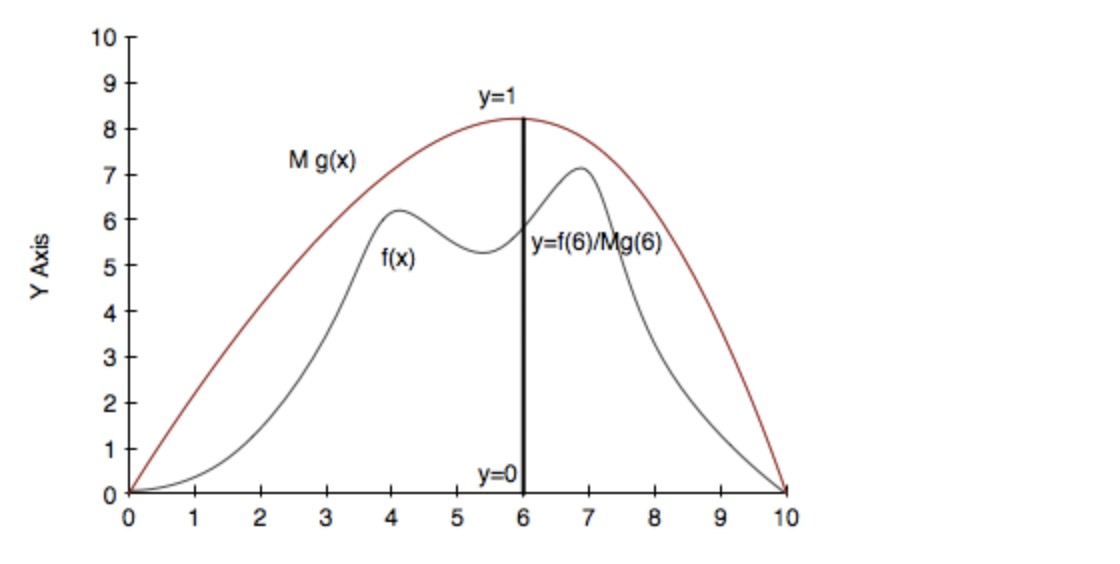Another way of seeing this whole thing is that we will draw the sample from a proposal distribution and re-weight the integral appropriately so that the expectation with respect to the correct distribution is used. And since $\frac{f}{g}$ is flatter than $f$, the variance of $h\times\frac{f}{g}$ is smaller that the variance of $h\times f$ and therefore the error will be smaller for all N.

### Code of Important Sampling ( Example: $\int_{0}^{\pi}sin(x)xdx$ )


from scipy import stats
from scipy.stats import norm

mu = 2;
sig = .7;

f = lambda x: np.sin(x)*x
infun = lambda x: np.sin(x)-x*np.cos(x)
p = lambda x: (1/np.sqrt(2*np.pi*sig**2))*np.exp(-(x-mu)**2/(2.0*sig**2))
normfun = lambda x: norm.cdf(x-mu, scale=sig)

# Range of integration
xmax = np.pi
xmin = 0

# Number of draws
N = 1000

#Just Want to plot the function
x = np.linspace(xmin,xmax,1000)
plt.figure(figsize=(18,8))
plt.subplot(1,2,1)
plt.plot(x, f(x), 'b', label='Original $x\sin(x)$')
plt.plot(x, p(x), 'r', label='Important Sampling Function:')
plt.plot(x, np.ones(1000)/np.pi, 'k')
xis = mu + sig*np.random.randn(N,1)
plt.plot(xis, 1/(np.pi*p(xis)), '.', alpha=0.1)
plt.xlim([0, np.pi])
plt.ylim([0, 2])
plt.xlabel('x')
plt.legend()

## VANILLA MONTE CAROL
Ivmc = np.zeros(1000)
for k in np.arange(0, 1000):
x = np.random.uniform(low=xmin, high=xmax, size=N)
Ivmc[k] = (xmax-xmin)*np.mean(f(x))

print('Mean basic MC estimate:', np.mean(Ivmc))
print('Standard deviation of our estimates:', np.std(Ivmc))

## IMPORTANCE SAMPLING, choose gaussian so it is
## similar to the original functions

Iis = np.zeros(1000)
for k in np.arange(0, 1000):
xis = mu + sig * np.random.randn(N,1)
xis = xis[(xis<xmax) & (xis>xmin)]

# normalization for gaussian from 0 to pi
normal = normfun(np.pi)-normfun(0)
Iis[k] = np.mean(f(xis)/p(xis))*normal

print('Mean important sampling MC estimate:', np.mean(Iis))
print('Standard deviation of our estimates:', np.std(Iis))

plt.subplot(1,2,2)
plt.hist(Iis, 30, histtype='step', label='Importance Sampling')
plt.hist(Ivmc, 30, color='r', histtype='step', label='Vanilla')
plt.grid('on')
plt.legend()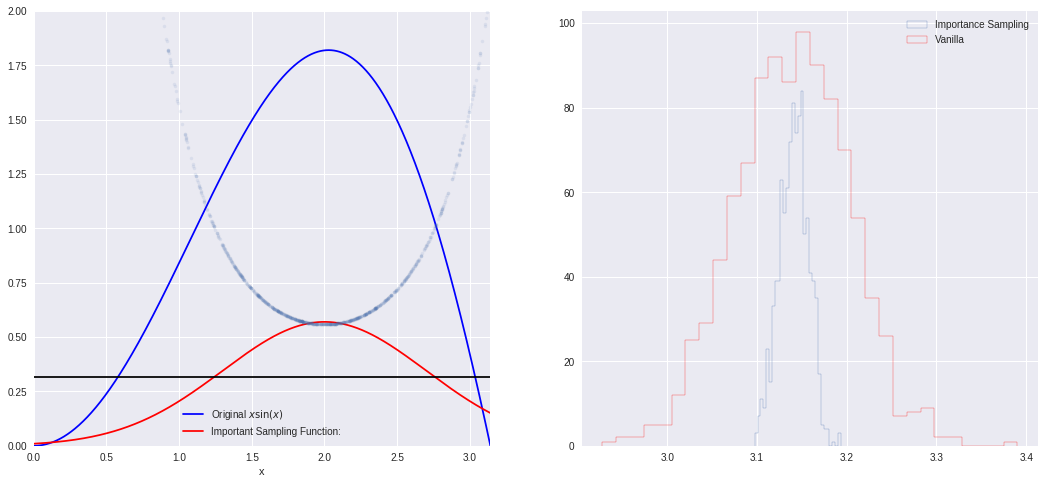## 4. Partical Filter

Particle filter uses the re-sampleing idea to very efficient and elegant sequential inference tasks. The goal here if to make a fast sampling based inference algorithm fot the state sapce model.

Kalman Filter (KF) assume the transition model and transmission model are under linear gaussian system. However, KF is required to implement in high dimensional spaces or when the transition model is not Gaussian. Sampling-based algorithm can be useful. The goal is to get samples from the posterior $p(X_{t}\vert Y_{1:t}) using the weighted re-sampling approach. We establish the recursive formulation like in the KF algorithm. We want to update the posterior of the hidden variables in light of each new observation. This is essentially a recursive two step process: 1. The starting point at time$t$is$p(X_{t}\vert Y_{1:t})=p(X_{t}\vert Y_{t},Y_{1:t-1})=\frac{p(X_{t}\vert Y_{1:t-1})p(Y_{t}\vert X_{t})}{\int p(X_{t}\vert Y_{1:t-1})p(Y_{t}\vert X_{t})\mathrm{d}X_{t}}$2. We want to draw sample from$p(X_{t}\vert Y_{1:t-1})$(treat like our proposal distribution for$p(X_{t}\vert Y_{1:t})$and give them weights:$w_{t}^{m}=\frac{p(Y_{t}\vert x_{t}^{m})}{\sum_{m=1}^{M}p(Y_{t}\vert X_{t}^{m})}$. We can now represent$p(X_{t}\vert Y_{1:t})$as the set of samples and weights, noting that$\sum_{m}w_{m}=1$. 3. To find weights and samples at the next time step for$p(X_{t+1}\vert Y_{1:t+1}), we do a time update and then a measurement update.

Time update: We decompose the probability into a term that we can replace with our sample representation and the transition probability:

The final term is the weighted sum of the transition probabilities conditioning on the samples of the previous states. This is a mixture distribution and samples can be easily drawn by choosing a component $m$ with probability $w^{m}$ and then drawing a sample from the relevant component. We again draw a set of samples and corresponding weights.

Measurement Update: Here we essentially perform step 1 again except now using the sample representation generated in the Time Update. We have a new observation $Y_{t+1}$ which we use to generate new weights for the Time Update in the next step.

Trading the Time and Measurement updates, we can proceed sequentially down the chain. The following schematic illustrates the process where the mixture distribution for the posterior at each time step is represented by circles whose sizes indicate their weights.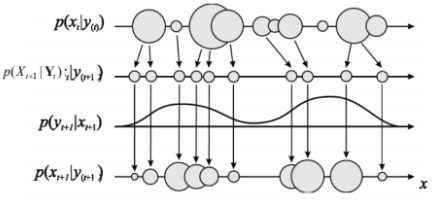Particle Filters are especially interesting because we can now draw samples from more complicated distributions such as SSMs.